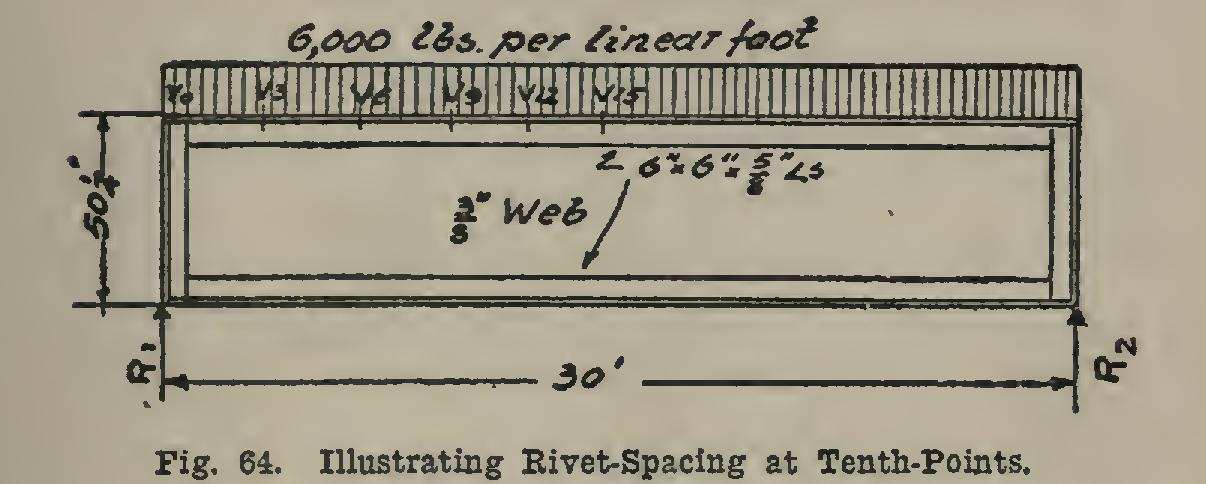# Girders 40

## pounds, spacing, loads and bridge

Page: 1 2 3 4 5 6 7

As an example, let it be required to determine the rivet-spacing at the end and at points sep arated a distance equal to one-tenth the deck plate-girder span of Fig. 64. This would require the spacing to be found at the end, and at 3, 6, 9, 12, and 15 feet from the end. Of course the rivet spacing on the right side of the middle of the girder is the same as that as computed for the left side. The reaction is: pounds.The shears (see page 172 for method of com putation) are: = 90,000-0 = 90,000 pounds.

= 90,000Ś 3x6,000 = 72,000 pounds.

= 90,000Ś 6x6,000 = 54,000 pounds.

= 90,000Ś 9x6,000 = 36,000 pounds. 90,000-12x6,000 = 18,000 pounds.

Vä-= 90,000-15x6,000 = 0 pounds.

If the allowable unit-stress in bearing is taken as 12,000 pounds per square inch, the value of a rivet in a web is (C 195) 3,940 pounds. The rivet-spacings computed by means of the formula =(3,940x43.25)+90,000 = 1.89 in., say 11 in. =(3,940x43.25)+72,000 = 2.37 in., say 21 in. s, =(3,940x43.25)+54,000 = 3.16 in., say 3 in. =(3,940x43.25)+36,000 = 4.73 in., say 41 in. sä=(3,940x43.25)+18,000 = 9.47 in., say 911 in.

= infinitely great.

This spacing should be decreased inch, since the load rests directly upon the flanges (see page 227). The spacing used would be: From end to 3-foot section, use 11-inch spacing From 3 to 6-foot section, use 11-inch spacing From 6 to 9-foot section, use 2k-inch spacing From 9 to 12-foot section, use 41-inch spacing From 12 to 15-foot section, use 6 -inch spacing 43. Engine and Equivalent Loads. For any

particular plate-girder span, the maximum shear and maximum moments due to any of the stand and locomotives may be obtained from tables given in the specifications for railroad bridges. In order to determine the stresses in bridges under locomotive (wheel) loads, the actual com putations must be performed for each particular bridge; and different computations are required for the several members of any given bridge, since the locomotive must be in different posi tions to give the greatest stresses in different members.

It is much easier to work out the stresses in a bridge under uniform load than under loco motive loads, and so most specifications give what are known as equivalent uniform loads. These are the uniform loads which will give the same shear and same moment (as far as prac tical use is concerned) as the locomotive for which they are given. These equivalent loads are then put over the bridge, and the stresses readily determined. This matter will be more fully explained in our treatment of Stresses in Bridge Trusses.

Page: 1 2 3 4 5 6 7